﻿ Monitoring Parkinson's Disease | Example | Neural Designer

# Telemonitoring of Parkinson's disease progression by non-invasive speech tests

Parkinson's disease is a degenerative disorder of the central nervous system.

There is no cure for this disease, but medications, surgery, and multidisciplinary management can relieve the symptoms.

The stage of the disease determines what group of drugs is most beneficial to relieve the symptoms.

This study aims to predict the clinician's Parkinson's disease symptom score on the Unified Parkinson's Disease Rating Scale (UPDRS) scale.### Contents:

This example is solved with Neural Designer. To follow it step by step, you can use the free trial.

## 1. Application type

The predicted variables are continuous (motor UPDRS and total UPDRS). This is an approximation project.

## 2. Data set

This dataset is composed of a range of biomedical voice measurements from 42 people with early-stage Parkinson's disease. They were recruited to a six-month trial of a telemonitoring device for remote symptom progression monitoring. The recordings were automatically captured in the patient's homes.

The following table depicts the names, descriptions, and uses of all the variables in the data set.

• subject - Integer that uniquely identifies each subject.
• age - Subject age.
• sex - Subject gender '0' - male, '1' - female.
• test_time - Time since recruitment into the trial. The integer part is the number of days since recruitment.
• Jitter(%), Jitter(Abs), Jitter:RAP, Jitter:PPQ5, Jitter:DDP - Several measures of variation in fundamental frequency.
• Shimmer, Shimmer(dB), Shimmer:APQ3, Shimmer:APQ5, Shimmer:APQ11, Shimmer:DDA - Several measures of variation in amplitude.
• NHR,HNR - Two measures of the ratio of noise to tonal components in the voice.
• RPDE - A nonlinear dynamical complexity measure.
• DFA - Signal fractal scaling exponent.
• PPE - A nonlinear measure of fundamental frequency variation.
• motor_UPDRS - Clinician's motor Unified Parkinson's Disease Rating Scale (UPDRS) score, linearly interpolated.
• total_UPDRS - Clinician's total Unified Parkinson's Disease Rating Scale (UPDRS) score, linearly interpolated.

In this case, the number of variables is 22, and the number of instances is 5875. We will be using 19 since the subject is just an identifier. 17 of which input variables and 2 are target variables(motor and total UPDRS).

There are 3525 instances for training (60%), 1175 instances for generalization (20%), 1175 instances for testing (20%).

Calculating the data distributions helps us to check for the correctness of the available information and detect anomalies. The following charts show the histograms for the variables motor_UPDRS and total_UPDRS.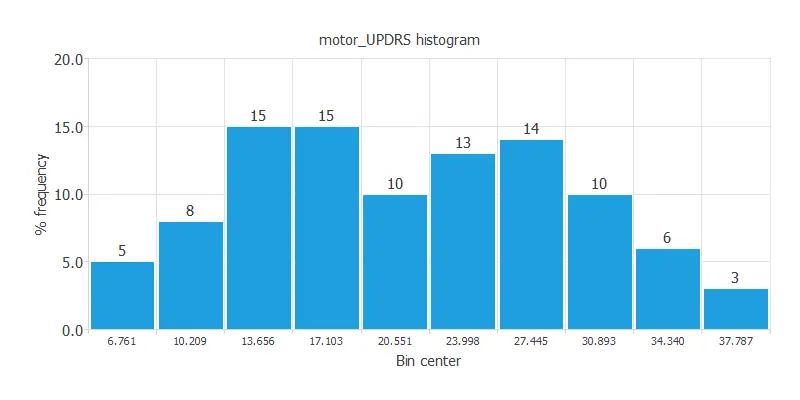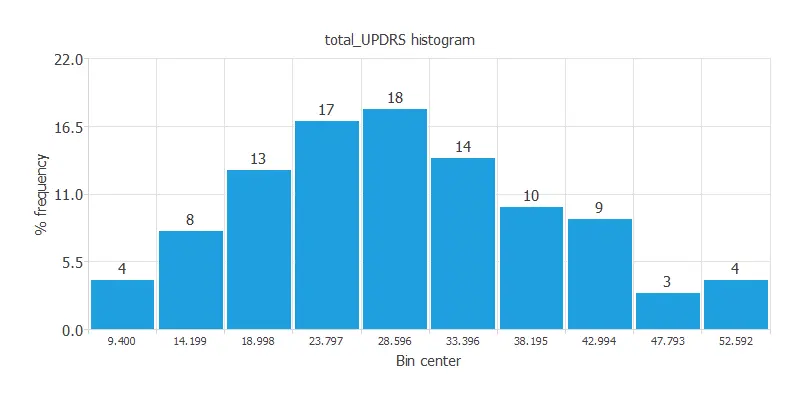As we can see, both target variables present a normal distribution. This information will later be used to define the neural network parameters and when choosing the training strategy.

## 3. Neural network

The second step is to set the model parameters. For approximation project type it is composed of:

• Scaling layer.
• Perceptron layers.
• Unscaling layer.

The Mean and standard deviation scaling method is set as the scaling method, while the minimum and maximum unscaling method is set as the unscaling method. The activation function chosen for this model are the hyperbolic tangent activation function and the linear activation function for the hidden and the output layers, respectively.

A graphical representation of the neural network is depicted next. It contains a scaling layer, 2 perceptron layers, and an unscaling layer. The number of inputs is 19, and the number of outputs is 2. The complexity, represented by the numbers of neurons in the hidden layer is 3.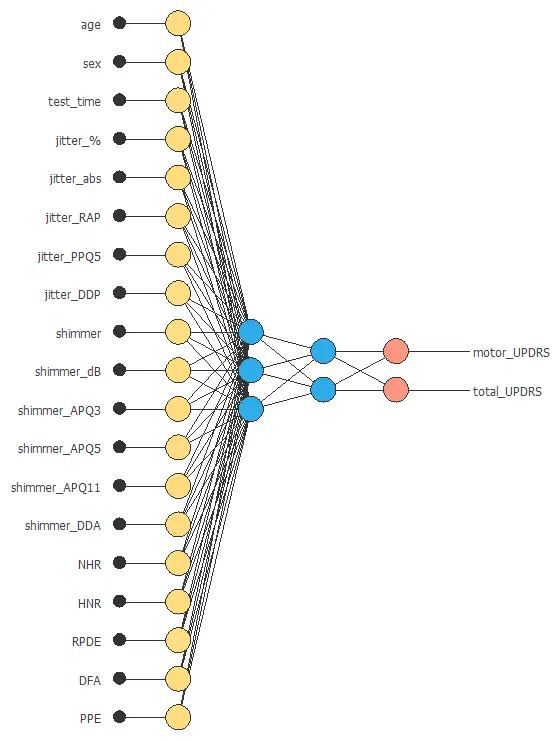## 4. Training strategy

The fourth step is to select an appropriate training strategy. It is composed of two things:

• A loss index.
• An optimization algorithm.

The loss index defines what the neural network will learn. It is composed of an error term and a regularization term.

The normalized squared error is used here as the error term, while the regularization term used is the L2 regularization. We use a weak weight for this regularization term.

The optimization algorithm is in charge of searching for the neural network parameters that minimize the loss index. Here we chose the quasi-Newton method as optimization algorithm.

The following chart shows how the error decreases with the iterations during the training process.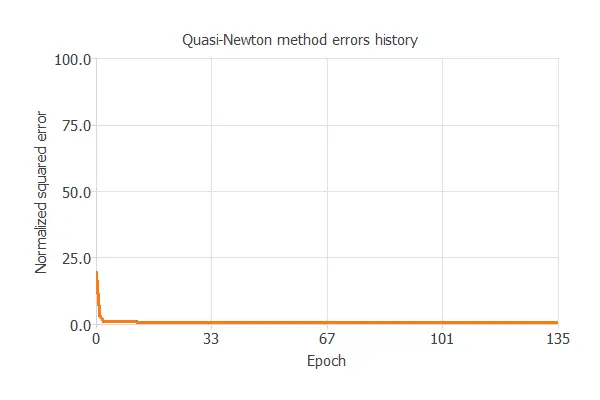The final values are training error = 0.565 NSE and selection error = 0.588 NSE.

## 5. Model selection

The objective of model selection is to find the network architecture with the best generalization properties, that is, that which minimizes the error on the selected instances of the data set.

More specifically, we want to find a neural network with a selection error of less than 0.426 NSE, which is the value that we have achieved so far.

Order selection algorithms train several network architectures with a different number of neurons and select that with the smallest selection error.

The incremental order method starts with a small number of neurons and increases the complexity at each iteration. The following chart shows the training error (blue) and the selection error (orange) as a function of the number of neurons.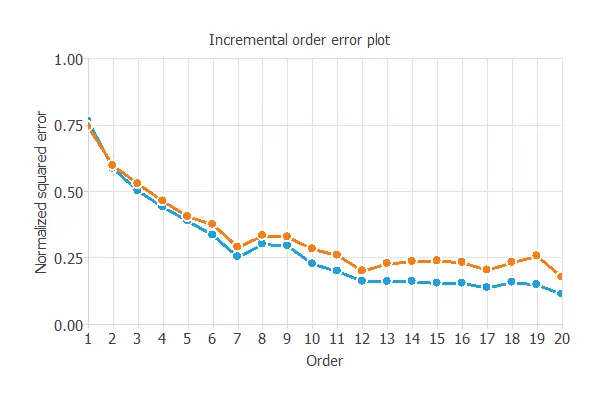The final selection error achieved is 0.176 for an optimal number of neurons of 20.The graph above represents the architecture of the final neural network.

## 6. Testing analysis

A standard method for testing the predictive model is to compare the outputs from the neural network against data never seen before.

The following images show the linear regression analysis for the two main outputs (motor_UPDRS and total_UPDRS).

The following chart illustrates the linear regression for the scaled output motor_UPDRS. The grey line would indicate a perfect fit.For this variable, there is a good correlation, with an R2 value of 0.898. Therefore, we can state that the neural network predicts well the variable motor_UPDRS.

The following chart illustrates the linear regression for the scaled output total_UPDRS. The grey line would indicate a perfect fit.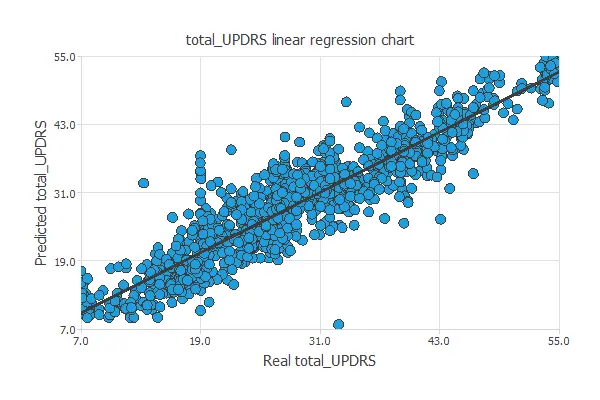As previously, we can observe a good correlation, being the value of R2 0.911. Again, we can state that the neural network predicts well the variable total_UPDRS.

## 7. Model deployment

The neural network is now ready to estimate the motor_UPDRS and the total_UPDRS with satisfactory quality over the same data range.

We can plot a directional output of the neural network to see hoy the total UPDRS and motor UPDRS varies with a given input, for all other inputs fixed. The following plot shows how the total UPDRS varies as a function of the shimer_dB.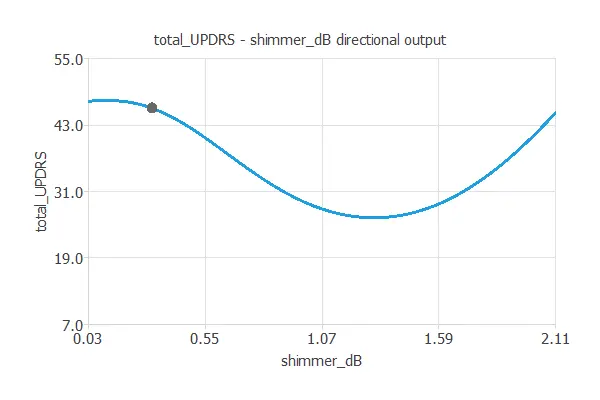Also, we can use the mathematical expression of the neural network, which is listed next.

```scaled_age = (age-64.8049)/8.82152;
scaled_sex = (sex-0.317787)/0.465656;
scaled_test_time = (test_time-92.8637)/53.4456;
scaled_jitter_% = (jitter_%-0.00615376)/0.00562423;
scaled_jitter_abs = (jitter_abs-4.40319e-05)/3.59827e-05;
scaled_jitter_RAP = (jitter_RAP-0.00298718)/0.00312382;
scaled_jitter_PPQ5 = (jitter_PPQ5-0.00327685)/0.00373154;
scaled_jitter_DDP = (jitter_DDP-0.00896168)/0.00937149;
scaled_shimmer = (shimmer-0.0340352)/0.0258352;
scaled_shimmer_dB = (shimmer_dB-0.31096)/0.230254;
scaled_shimmer_APQ3 = (shimmer_APQ3-0.0171557)/0.0132371;
scaled_shimmer_APQ5 = (shimmer_APQ5-0.0201442)/0.0166639;
scaled_shimmer_APQ11 = (shimmer_APQ11-0.0274809)/0.0199861;
scaled_shimmer_DDA = (shimmer_DDA-0.0514669)/0.0397114;
scaled_NHR = (NHR-0.0321198)/0.0596923;
scaled_HNR = (HNR-21.6795)/4.2911;
scaled_RPDE = (RPDE-0.541473)/0.100986;
scaled_DFA = (DFA-0.65324)/0.070902;
scaled_PPE = (PPE-0.219589)/0.0914985;
y_1_1 = tanh (-1.57187+ (scaled_age*0.0142681)+ (scaled_sex*0.199926)+ (scaled_test_time*-0.327582)+ (scaled_jitter_%*0.0144707)+ (scaled_jitter_abs*-0.263242)+ (scaled_jitter_RAP*0.234534)+ (scaled_jitter_PPQ5*-0.255756)+ (scaled_jitter_DDP*0.239377)+ (scaled_shimmer*-0.00978277)+ (scaled_shimmer_dB*-0.324869)+ (scaled_shimmer_APQ3*0.098755)+ (scaled_shimmer_APQ5*-1.39619)+ (scaled_shimmer_APQ11*0.981768)+ (scaled_shimmer_DDA*0.0968944)+ (scaled_NHR*-0.312164)+ (scaled_HNR*-0.0552177)+ (scaled_RPDE*0.267387)+ (scaled_DFA*0.550126)+ (scaled_PPE*-0.12346));
y_1_2 = tanh (-0.647599+ (scaled_age*0.363908)+ (scaled_sex*-0.558054)+ (scaled_test_time*0.610976)+ (scaled_jitter_%*-0.153809)+ (scaled_jitter_abs*-0.488345)+ (scaled_jitter_RAP*0.229893)+ (scaled_jitter_PPQ5*-0.0907269)+ (scaled_jitter_DDP*0.166763)+ (scaled_shimmer*0.195535)+ (scaled_shimmer_dB*0.427208)+ (scaled_shimmer_APQ3*-0.137411)+ (scaled_shimmer_APQ5*0.00229258)+ (scaled_shimmer_APQ11*-0.0306761)+ (scaled_shimmer_DDA*-0.151346)+ (scaled_NHR*-0.0278939)+ (scaled_HNR*-0.430714)+ (scaled_RPDE*-0.251164)+ (scaled_DFA*-0.207627)+ (scaled_PPE*-0.112005));
y_1_3 = tanh (1.34561+ (scaled_age*-2.01591)+ (scaled_sex*-1.45682)+ (scaled_test_time*-0.0643536)+ (scaled_jitter_%*-1.24657)+ (scaled_jitter_abs*1.95675)+ (scaled_jitter_RAP*-0.509131)+ (scaled_jitter_PPQ5*0.531695)+ (scaled_jitter_DDP*-0.51028)+ (scaled_shimmer*0.147611)+ (scaled_shimmer_dB*-0.280139)+ (scaled_shimmer_APQ3*-0.0145424)+ (scaled_shimmer_APQ5*0.111495)+ (scaled_shimmer_APQ11*-0.426834)+ (scaled_shimmer_DDA*-0.0197965)+ (scaled_NHR*-0.225547)+ (scaled_HNR*-0.560643)+ (scaled_RPDE*0.136243)+ (scaled_DFA*-0.622104)+ (scaled_PPE*-0.383443));
y_1_4 = tanh (0.274653+ (scaled_age*-1.39494)+ (scaled_sex*0.450681)+ (scaled_test_time*0.136394)+ (scaled_jitter_%*-0.286674)+ (scaled_jitter_abs*0.927021)+ (scaled_jitter_RAP*-0.370346)+ (scaled_jitter_PPQ5*0.207622)+ (scaled_jitter_DDP*-0.283957)+ (scaled_shimmer*0.0121324)+ (scaled_shimmer_dB*-0.209122)+ (scaled_shimmer_APQ3*0.0037933)+ (scaled_shimmer_APQ5*0.217412)+ (scaled_shimmer_APQ11*-0.259567)+ (scaled_shimmer_DDA*-0.000554402)+ (scaled_NHR*-0.16015)+ (scaled_HNR*-0.0817284)+ (scaled_RPDE*0.208028)+ (scaled_DFA*-0.116146)+ (scaled_PPE*-0.149016));
y_1_5 = tanh (-1.17566+ (scaled_age*2.73576)+ (scaled_sex*1.24121)+ (scaled_test_time*-0.018021)+ (scaled_jitter_%*0.398299)+ (scaled_jitter_abs*-0.603297)+ (scaled_jitter_RAP*0.136713)+ (scaled_jitter_PPQ5*-0.167916)+ (scaled_jitter_DDP*0.180086)+ (scaled_shimmer*-0.0622196)+ (scaled_shimmer_dB*0.104293)+ (scaled_shimmer_APQ3*0.0399341)+ (scaled_shimmer_APQ5*-0.189551)+ (scaled_shimmer_APQ11*0.166149)+ (scaled_shimmer_DDA*0.00915821)+ (scaled_NHR*0.091129)+ (scaled_HNR*0.192346)+ (scaled_RPDE*-0.0229184)+ (scaled_DFA*0.171639)+ (scaled_PPE*0.138643));
y_1_6 = tanh (-0.535227+ (scaled_age*-0.545172)+ (scaled_sex*-0.0112904)+ (scaled_test_time*0.204865)+ (scaled_jitter_%*0.212772)+ (scaled_jitter_abs*0.327968)+ (scaled_jitter_RAP*-0.202439)+ (scaled_jitter_PPQ5*0.103907)+ (scaled_jitter_DDP*-0.282324)+ (scaled_shimmer*0.136306)+ (scaled_shimmer_dB*-0.489712)+ (scaled_shimmer_APQ3*0.0687708)+ (scaled_shimmer_APQ5*-0.174426)+ (scaled_shimmer_APQ11*0.26662)+ (scaled_shimmer_DDA*0.0662447)+ (scaled_NHR*-0.255934)+ (scaled_HNR*0.0775486)+ (scaled_RPDE*0.302503)+ (scaled_DFA*0.441738)+ (scaled_PPE*-0.074675));
y_1_7 = tanh (0.260647+ (scaled_age*-0.305241)+ (scaled_sex*0.546527)+ (scaled_test_time*-0.415429)+ (scaled_jitter_%*-0.714838)+ (scaled_jitter_abs*-0.0625429)+ (scaled_jitter_RAP*0.272195)+ (scaled_jitter_PPQ5*0.438837)+ (scaled_jitter_DDP*0.217629)+ (scaled_shimmer*0.359828)+ (scaled_shimmer_dB*-0.290198)+ (scaled_shimmer_APQ3*-0.0277553)+ (scaled_shimmer_APQ5*-0.582877)+ (scaled_shimmer_APQ11*-0.00912689)+ (scaled_shimmer_DDA*-0.0180083)+ (scaled_NHR*0.0450529)+ (scaled_HNR*0.0991328)+ (scaled_RPDE*0.404628)+ (scaled_DFA*0.366001)+ (scaled_PPE*0.133061));
y_1_8 = tanh (1.14137+ (scaled_age*3.13393)+ (scaled_sex*1.83063)+ (scaled_test_time*0.0287885)+ (scaled_jitter_%*0.357391)+ (scaled_jitter_abs*0.0537099)+ (scaled_jitter_RAP*-0.142751)+ (scaled_jitter_PPQ5*-0.00404539)+ (scaled_jitter_DDP*-0.260003)+ (scaled_shimmer*-0.24376)+ (scaled_shimmer_dB*-0.166464)+ (scaled_shimmer_APQ3*-0.0517241)+ (scaled_shimmer_APQ5*0.676053)+ (scaled_shimmer_APQ11*-0.0482483)+ (scaled_shimmer_DDA*-0.0774931)+ (scaled_NHR*-0.141586)+ (scaled_HNR*-0.168477)+ (scaled_RPDE*-0.0109972)+ (scaled_DFA*0.234475)+ (scaled_PPE*-0.0274888));
y_1_9 = tanh (-2.74518+ (scaled_age*-6.00073)+ (scaled_sex*-1.50233)+ (scaled_test_time*-0.0392235)+ (scaled_jitter_%*-0.106941)+ (scaled_jitter_abs*-0.141498)+ (scaled_jitter_RAP*0.0395385)+ (scaled_jitter_PPQ5*0.222543)+ (scaled_jitter_DDP*-0.0251332)+ (scaled_shimmer*0.206609)+ (scaled_shimmer_dB*-0.067808)+ (scaled_shimmer_APQ3*-0.0360847)+ (scaled_shimmer_APQ5*-0.32473)+ (scaled_shimmer_APQ11*-0.0252375)+ (scaled_shimmer_DDA*-0.0397432)+ (scaled_NHR*-0.0134401)+ (scaled_HNR*-0.331094)+ (scaled_RPDE*0.0508102)+ (scaled_DFA*-0.286483)+ (scaled_PPE*-0.192009));
y_1_10 = tanh (-1.66639+ (scaled_age*5.82398)+ (scaled_sex*4.80633)+ (scaled_test_time*-0.0496743)+ (scaled_jitter_%*0.261225)+ (scaled_jitter_abs*-0.199432)+ (scaled_jitter_RAP*0.00552772)+ (scaled_jitter_PPQ5*-0.0664937)+ (scaled_jitter_DDP*-0.0210807)+ (scaled_shimmer*-0.0427847)+ (scaled_shimmer_dB*-0.00957307)+ (scaled_shimmer_APQ3*0.0417199)+ (scaled_shimmer_APQ5*-0.25159)+ (scaled_shimmer_APQ11*0.2216)+ (scaled_shimmer_DDA*0.0711813)+ (scaled_NHR*-0.018993)+ (scaled_HNR*0.314962)+ (scaled_RPDE*-0.0263577)+ (scaled_DFA*0.0664688)+ (scaled_PPE*0.208975));
y_1_11 = tanh (-0.947496+ (scaled_age*0.966098)+ (scaled_sex*-0.0558299)+ (scaled_test_time*0.293533)+ (scaled_jitter_%*-0.152948)+ (scaled_jitter_abs*-1.20488)+ (scaled_jitter_RAP*0.549669)+ (scaled_jitter_PPQ5*0.163803)+ (scaled_jitter_DDP*0.562747)+ (scaled_shimmer*0.593074)+ (scaled_shimmer_dB*0.137946)+ (scaled_shimmer_APQ3*-0.282452)+ (scaled_shimmer_APQ5*-0.0462759)+ (scaled_shimmer_APQ11*0.222792)+ (scaled_shimmer_DDA*-0.287875)+ (scaled_NHR*0.0924362)+ (scaled_HNR*0.108539)+ (scaled_RPDE*-0.00393671)+ (scaled_DFA*0.232951)+ (scaled_PPE*0.298073));
y_1_12 = tanh (-1.70765+ (scaled_age*-1.8023)+ (scaled_sex*0.292029)+ (scaled_test_time*0.12419)+ (scaled_jitter_%*-0.0334417)+ (scaled_jitter_abs*0.347251)+ (scaled_jitter_RAP*-0.179604)+ (scaled_jitter_PPQ5*-0.180554)+ (scaled_jitter_DDP*-0.10233)+ (scaled_shimmer*-0.437318)+ (scaled_shimmer_dB*-0.0040637)+ (scaled_shimmer_APQ3*0.0805409)+ (scaled_shimmer_APQ5*0.108444)+ (scaled_shimmer_APQ11*0.109846)+ (scaled_shimmer_DDA*0.0764655)+ (scaled_NHR*-0.145952)+ (scaled_HNR*-0.0984104)+ (scaled_RPDE*0.177627)+ (scaled_DFA*0.276637)+ (scaled_PPE*0.0799496));
y_1_13 = tanh (0.0570207+ (scaled_age*0.762764)+ (scaled_sex*0.115528)+ (scaled_test_time*0.304763)+ (scaled_jitter_%*0.222103)+ (scaled_jitter_abs*0.671626)+ (scaled_jitter_RAP*-0.492379)+ (scaled_jitter_PPQ5*0.777221)+ (scaled_jitter_DDP*-0.393744)+ (scaled_shimmer*0.0546041)+ (scaled_shimmer_dB*-0.397712)+ (scaled_shimmer_APQ3*0.133778)+ (scaled_shimmer_APQ5*-0.460625)+ (scaled_shimmer_APQ11*0.370331)+ (scaled_shimmer_DDA*0.124102)+ (scaled_NHR*-0.251188)+ (scaled_HNR*0.384866)+ (scaled_RPDE*-0.328552)+ (scaled_DFA*-0.198296)+ (scaled_PPE*0.0423754));
y_1_14 = tanh (0.742988+ (scaled_age*0.191191)+ (scaled_sex*0.545352)+ (scaled_test_time*-0.196096)+ (scaled_jitter_%*-0.898267)+ (scaled_jitter_abs*0.307062)+ (scaled_jitter_RAP*0.219658)+ (scaled_jitter_PPQ5*-0.300967)+ (scaled_jitter_DDP*0.266665)+ (scaled_shimmer*0.360872)+ (scaled_shimmer_dB*0.266681)+ (scaled_shimmer_APQ3*-0.398673)+ (scaled_shimmer_APQ5*0.316689)+ (scaled_shimmer_APQ11*-0.327585)+ (scaled_shimmer_DDA*-0.39974)+ (scaled_NHR*0.0277494)+ (scaled_HNR*-0.684486)+ (scaled_RPDE*0.355562)+ (scaled_DFA*0.672872)+ (scaled_PPE*-0.352162));
y_1_15 = tanh (-2.98768+ (scaled_age*-9.05004)+ (scaled_sex*-5.00092)+ (scaled_test_time*-0.0450689)+ (scaled_jitter_%*-0.556879)+ (scaled_jitter_abs*0.686268)+ (scaled_jitter_RAP*-0.110226)+ (scaled_jitter_PPQ5*0.132499)+ (scaled_jitter_DDP*-0.139117)+ (scaled_shimmer*0.332987)+ (scaled_shimmer_dB*-0.0524302)+ (scaled_shimmer_APQ3*-0.0216971)+ (scaled_shimmer_APQ5*-0.022606)+ (scaled_shimmer_APQ11*-0.125182)+ (scaled_shimmer_DDA*-0.0548148)+ (scaled_NHR*-0.0501857)+ (scaled_HNR*-0.0672972)+ (scaled_RPDE*-0.0709088)+ (scaled_DFA*-0.247622)+ (scaled_PPE*-0.102323));
y_1_16 = tanh (0.525004+ (scaled_age*3.77077)+ (scaled_sex*0.418377)+ (scaled_test_time*0.0143505)+ (scaled_jitter_%*0.169533)+ (scaled_jitter_abs*-0.387627)+ (scaled_jitter_RAP*0.209145)+ (scaled_jitter_PPQ5*-0.00518281)+ (scaled_jitter_DDP*0.0881353)+ (scaled_shimmer*-0.115382)+ (scaled_shimmer_dB*0.0410955)+ (scaled_shimmer_APQ3*0.0603984)+ (scaled_shimmer_APQ5*-0.24177)+ (scaled_shimmer_APQ11*0.14996)+ (scaled_shimmer_DDA*0.0160391)+ (scaled_NHR*0.0431122)+ (scaled_HNR*0.182838)+ (scaled_RPDE*0.00320457)+ (scaled_DFA*0.0584272)+ (scaled_PPE*0.116415));
y_1_17 = tanh (-1.72712+ (scaled_age*-2.85359)+ (scaled_sex*0.468617)+ (scaled_test_time*-0.0916411)+ (scaled_jitter_%*0.466822)+ (scaled_jitter_abs*0.42038)+ (scaled_jitter_RAP*-0.316034)+ (scaled_jitter_PPQ5*-0.259823)+ (scaled_jitter_DDP*-0.395119)+ (scaled_shimmer*0.232158)+ (scaled_shimmer_dB*-0.0940998)+ (scaled_shimmer_APQ3*-0.0423788)+ (scaled_shimmer_APQ5*0.218758)+ (scaled_shimmer_APQ11*-0.0369179)+ (scaled_shimmer_DDA*-0.0452157)+ (scaled_NHR*-0.021343)+ (scaled_HNR*-0.0451856)+ (scaled_RPDE*-0.0152138)+ (scaled_DFA*0.269252)+ (scaled_PPE*0.0137743));
y_1_18 = tanh (-0.831512+ (scaled_age*-0.163493)+ (scaled_sex*-0.44628)+ (scaled_test_time*0.0460487)+ (scaled_jitter_%*0.813914)+ (scaled_jitter_abs*-0.987557)+ (scaled_jitter_RAP*0.116509)+ (scaled_jitter_PPQ5*-0.268832)+ (scaled_jitter_DDP*0.185493)+ (scaled_shimmer*-0.0901849)+ (scaled_shimmer_dB*0.00625413)+ (scaled_shimmer_APQ3*0.257923)+ (scaled_shimmer_APQ5*-0.514261)+ (scaled_shimmer_APQ11*0.237781)+ (scaled_shimmer_DDA*0.278408)+ (scaled_NHR*0.143011)+ (scaled_HNR*0.440839)+ (scaled_RPDE*-0.337503)+ (scaled_DFA*-0.43974)+ (scaled_PPE*0.267874));
y_1_19 = tanh (0.177067+ (scaled_age*1.03567)+ (scaled_sex*-0.375498)+ (scaled_test_time*0.203699)+ (scaled_jitter_%*0.0532981)+ (scaled_jitter_abs*0.587539)+ (scaled_jitter_RAP*-0.207955)+ (scaled_jitter_PPQ5*0.148684)+ (scaled_jitter_DDP*-0.276378)+ (scaled_shimmer*-0.628715)+ (scaled_shimmer_dB*0.0894913)+ (scaled_shimmer_APQ3*0.199036)+ (scaled_shimmer_APQ5*-0.235172)+ (scaled_shimmer_APQ11*0.303011)+ (scaled_shimmer_DDA*0.189902)+ (scaled_NHR*0.0370405)+ (scaled_HNR*0.352241)+ (scaled_RPDE*-0.214757)+ (scaled_DFA*-0.330812)+ (scaled_PPE*-0.0289168));
y_1_20 = tanh (-0.250278+ (scaled_age*-0.994701)+ (scaled_sex*-2.35086)+ (scaled_test_time*0.208188)+ (scaled_jitter_%*-0.25914)+ (scaled_jitter_abs*1.09507)+ (scaled_jitter_RAP*-0.466343)+ (scaled_jitter_PPQ5*0.0732063)+ (scaled_jitter_DDP*-0.463876)+ (scaled_shimmer*-0.966316)+ (scaled_shimmer_dB*-0.139335)+ (scaled_shimmer_APQ3*0.157339)+ (scaled_shimmer_APQ5*0.975961)+ (scaled_shimmer_APQ11*-0.163554)+ (scaled_shimmer_DDA*0.148678)+ (scaled_NHR*-0.143984)+ (scaled_HNR*-0.0381107)+ (scaled_RPDE*0.0597062)+ (scaled_DFA*-0.0530085)+ (scaled_PPE*-0.0222058));
scaled_motor_UPDRS =  (0.453404+ (y_1_1*0.5553)+ (y_1_2*-0.574177)+ (y_1_3*0.776725)+ (y_1_4*1.09966)+ (y_1_5*3.72156)+ (y_1_6*-0.630386)+ (y_1_7*-0.559572)+ (y_1_8*-1.79982)+ (y_1_9*-1.55315)+ (y_1_10*-1.85222)+ (y_1_11*0.58258)+ (y_1_12*1.04273)+ (y_1_13*0.58985)+ (y_1_14*0.668582)+ (y_1_15*-2.63272)+ (y_1_16*-2.91211)+ (y_1_17*-1.05801)+ (y_1_18*0.782151)+ (y_1_19*-0.696425)+ (y_1_20*0.692395));
scaled_total_UPDRS =  (0.251572+ (y_1_1*0.460416)+ (y_1_2*-0.397904)+ (y_1_3*0.785814)+ (y_1_4*0.953163)+ (y_1_5*3.88818)+ (y_1_6*-0.685697)+ (y_1_7*-0.318125)+ (y_1_8*-1.70846)+ (y_1_9*-1.79835)+ (y_1_10*-2.00443)+ (y_1_11*0.446086)+ (y_1_12*1.11303)+ (y_1_13*0.573548)+ (y_1_14*0.544474)+ (y_1_15*-2.59489)+ (y_1_16*-3.29633)+ (y_1_17*-1.03204)+ (y_1_18*0.553401)+ (y_1_19*-0.523331)+ (y_1_20*0.689788));
(motor_UPDRS,total_UPDRS) = (0.5*(scaled_motor_UPDRS+1.0)*(39.511-5.0377)+5.0377,0.5*(scaled_total_UPDRS+1.0)*(54.992-7)+7);
```

The previous mathematical expression can be used in any medical software to predict the values of these parameters for a determined patient.

## References

• UCI Machine Learning Repository. Parkinson's Telemonitoring Data Set .
• A Tsanas, MA Little, PE McSharry, LO Ramig (2009). Accurate telemonitoring of Parkinson's disease progression by non-invasive speech tests, IEEE Transactions on Biomedical Engineering (to appear).